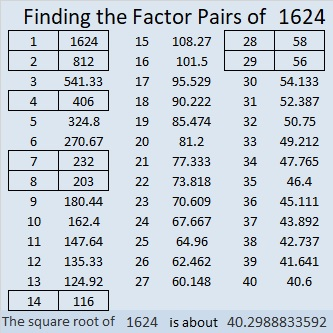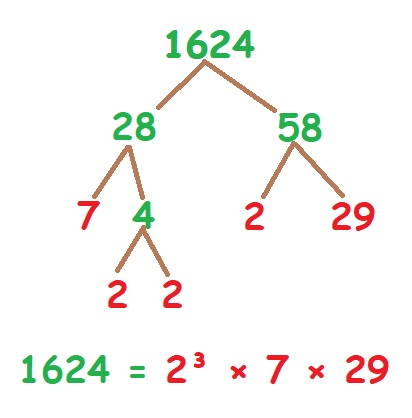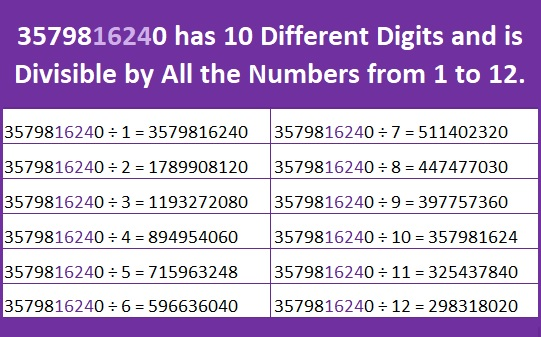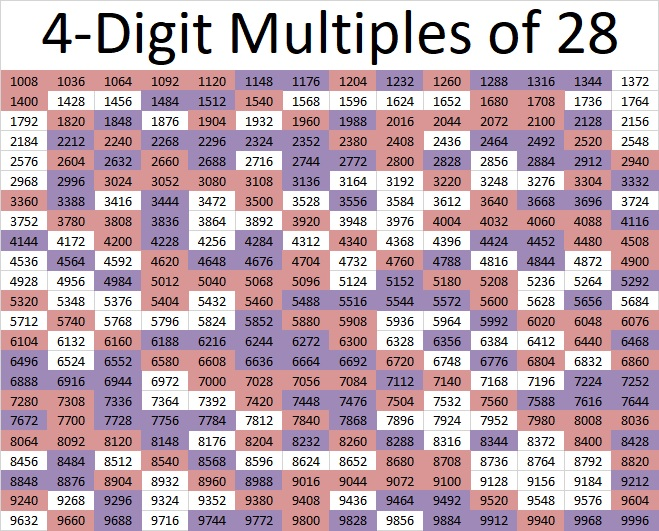# 1624 Applying Twelve Divisibility Rules to Permutations of 1234567890

Contents

### Today’s Puzzle:

Can you use divisibility rules to find a number that uses all ten digits exactly once and is divisible by all the numbers from 1 to 10?”

EVERY such number will be divisible by 1, of course. That’s the divisibility rule for 1. That one was super easy.

But guess what! No matter how you arrange those ten digits it will be divisible by 3 and by 9. Why? Because the sum of the digits of 1234567890 is 45, a number divisible by both 3 and 9. That’s the divisibility rule for 3 and for 9.

If the number you create ends with a zero, it will also be divisible by 5 and 10. That’s the divisibility rule for 5 and the divisibility rule for 10. AND it will also be divisible by 2 and by 6 because those are the divisibility rules for even numbers and for even numbers divisible by 3.

That leaves only three divisors to worry about: 4, 7, and 8. This post will talk about an easy and fun way to deal with those divisibility rules!

Did you know that no matter how many digits a number has, if the last digit is 2 or 6 and is preceded by an odd number, then that number will be divisible by 4? Also if the last digit of that number is 0, 4, or 8 and is preceded by an even number, then that number will be divisible by 4 as well? That’s a divisibility rule for 4.

If that number happens to have nine digits and we multiply it by 10, then the new product will also be divisible by 8. That’s because of the divisibility rule for 4 and the number getting multiplied by 2 × 5.

So 7 is the only divisor that is making any real trouble for us! Here’s how we will deal with division by 7: We will separate our number into smaller parts, all of which are divisible by 7. It would be easiest to separate it into three 3-digit numbers and then have those 9-digits be followed by a zero.

### 3-Digit Multiples of 7:

Now in the chart below we have all of the 3-digit multiples of 7. I have colored in all the numbers with a zero, and all numbers that repeat any of their own digits. We won’t be using any of the numbers that have been shaded in. However, we want to use at least one of the numbers printed in green. In fact, a green number should be your first choice because it is divisible by 4, while your second and third choices will be numbers that have not been shaded in.

And just like that, you can take ANY two numbers from the chart followed by a green number and zero, and you will have a number that is divisible by all the numbers from 1 to 10.If you take care that none of the digits repeat, which is very likely with so many choices, it will also be a number that has each of the ten digits exactly one time!

For example, if I choose 812 as my green number, and 357 as my second number, my leftover digits are 9-4-6. I can check all permutations of 9-4-6 to see if any of them are on the chart, and I notice that 469 is there so I form the number 3574698120. Here’s proof that it is divisible by every number from 1 to 10:Now you pick three of your own 3-digit numbers, attach a zero, and see how it does!

### Let’s Explore the 11-and 12-Divisibility Rules:

My chosen number is also divisible by 12 because it satisfies the 3- and the 4- divisibility rules. Is it divisible by 11?

3574698120 is NOT divisible by 11. I know that because the sum of the red numbers, 26, minus the sum of the blue numbers, 19, is 7 which is not a multiple of 11.

However, if I switch the 357 with the 469, my number still satisfies all the previous divisibility rules, but now 4693578120 has an additional factor! The sum of its red numbers, 28, minus the sum of its blue numbers, 17, is 11, clearly a multiple of 11.

The secret to having the bonus that your 10-digit number is divisible by 11, is having every other number add up to either 17 or 28. Those are the sums we should look for because 28 + 17 = 45, the total of all the digits, and 28 – 17 = 11, the difference satisfying the 11 divisibility rule.

Thus, 4693578120 is divisible by all the numbers from 1 to 12. Here’s a table showing all those divisions:Now you give it a try! Can you also use the 3-digit multiples of 7 Chart to find your own 10-digit number that is divisible by all the numbers from 1 to 12? Try it! I think you will have fun solving this puzzle, even if you have to try several different sets of 3-digit numbers to find one that works.

### Factors of 1624:

• 1624 is a composite number.
• Prime factorization: 1624 = 2 × 2 × 2 × 7 × 29, which can be written 1624 = 2³ × 7 × 29.
• 1624 has at least one exponent greater than 1 in its prime factorization so √1624 can be simplified. Taking the factor pair from the factor pair table below with the largest square number factor, we get √1624 = (√4)(√406) = 2√406.
• The exponents in the prime factorization are 3,1 and 1. Adding one to each exponent and multiplying we get (3 + 1)(1 + 1) (1 + 1) = 4 × 2 × 2 = 16. Therefore 1624 has exactly 16 factors.
• The factors of 1624 are outlined with their factor pair partners in the graphic below.### Factor Tree for 1624:

There are several possible factor trees for 1624. Here’s one of them.That one used the factor pair from 28 × 58.

### Wait a Minute!

1624 has all different digits and is divisible by 4 and by 7… Does that mean we can use it to form a number with 10 different digits that will be divisible by all the numbers from 1 to 10??!!!

Yes, we can. In fact, in this case, I was able to determine the needed multiples of 7 in my head because they were all 1- or 2-digit multiples!

Here’s what I did: 1624, is divisible by both 4 and 7, so 16240 is divisible by 4, 7, and 8. The missing digits are 3, 5, 7, 8, and 9. What multiples of 7 use just those digits? How about 7, 35, and 98?! Easy Peasy!

Those ten digits can be arranged in six different ways all of which are divisible by all the numbers from 1 to 10:
7359816240,
7983516240,
3579816240,
3598716240,
9873516240, and
9835716240.

Are any of those permutations of 1234567890 also divisible by 11? Yes!!! I used pencil and paper to make sure every other digit of my chosen10-digit number added up to 28 or 17. Here are all those divisions:It is also divisible by 13, 14, 15, and 16, but I didn’t use a divisibility rule for 13, so I didn’t include any of those divisors in the chart.

### 4-Digit Multiples of 28:

Here is a chart showing all the 4-digit multiples of 28. Numbers containing zero or a repeated digit have been shaded. Use the chart to pick a 4-digit number that can be placed before the zero in your 10-digit number. Then find 1-, 2-, or 3-digit multiples of 7 to make your own 10-digit number that uses all the digits and is divisible by all the numbers from 1 to 10. You might even be able to satisfy the 11-divisibility rule so that your number is divisible by every number from 1 to 12. Make sure you are having fun working on this problem!After solving the puzzle in several different ways, you might want to explore this puzzle that Andy Parkinson posted on Twitter:Since 4967 is a relatively large prime number, 480 Factors likely can be beaten. Maybe YOU will be the one who finds a 10-different-10-digit number with more factors!

### More about the Number 1624:

1624 is the hypotenuse of a Pythagorean triple:
1120-1176-1624, which is 56 times (20-21-29).

2(28)(29) = 1624. That makes 1624 four times the 28th triangular number.

This site uses Akismet to reduce spam. Learn how your comment data is processed.